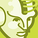# QlikView App Dev

Discussion Board for collaboration related to QlikView App Development.

cancel
Showing results for
Did you mean:Contributor

## Conditional color for Calculated dimension

Hi All,

i have Calculated Dimension like Below:

=Num(Aggr(Count(DISTINCT{<Bucket={On_Time}>}Employee_Number)/Sum(Aggr(Count(DISTINCT Employee_Number), Org_Hier.ORGANIZATION, Bucket, MonthBucket)), MonthBucket),'#,##0.00%')

i want to show Conditional color based on above dimension.

Example:

If (Compliance %) is   <=90   Red

>=91 and <=94   Yellow

95 and above Green

I have tried below expression but not working.

=if(num(Aggr(sum(aggr(Count(DISTINCT{<Status={Ontime}>}Employee_Number),[Updated Location],Region_Name,Site_Name, Status,Complaince_Training_Program, MonthBucket))/
Sum(Aggr(Count(DISTINCT Employee_Number),[Updated Location],Region_Name,Site_Name, Status,Complaince_Training_Program, MonthBucket)), MonthBucket),'##,#0%') <=90,RGB(255,0,0),Yellow())

How to achieve in Calculated Dimension,

Any Help would be greatly appreciated.

Thanks

Joshi

5 RepliesMVP

May be try this

If(Aggr(Count(DISTINCT{<Bucket={On_Time}>}Employee_Number)/Sum(Aggr(Count(DISTINCT Employee_Number), Org_Hier.ORGANIZATION, Bucket, MonthBucket)), MonthBucket) <= 0.90, RGB(255,0,0),

If(Aggr(Count(DISTINCT{<Bucket={On_Time}>}Employee_Number)/Sum(Aggr(Count(DISTINCT Employee_Number), Org_Hier.ORGANIZATION, Bucket, MonthBucket)), MonthBucket) <= 0.94, Yellow(), Green()))Contributor
Author

Hi Sunny,

No Luck only else part showing Green Color.

Thanks,MVP

Can you try this?

If(Count(DISTINCT{<Bucket={On_Time}>}Employee_Number)/Sum(Aggr(Count(DISTINCT Employee_Number), Org_Hier.ORGANIZATION, Bucket, MonthBucket)) <= 0.90, RGB(255,0,0),

If(Count(DISTINCT{<Bucket={On_Time}>}Employee_Number)/Sum(Aggr(Count(DISTINCT Employee_Number), Org_Hier.ORGANIZATION, Bucket, MonthBucket)) <= 0.94, Yellow(), Green()))Contributor
Author

Hi Sunny,

Still no Luck

Thanks,

joshiMVP

Would you be able to share a sample?Community Browser